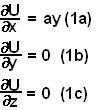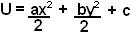## Saturday, May 3, 2008

### Irodov Problem 1.133

Force is the negative gradient of potential i.e. in catersian coordinates we have,.

In this problem the question is whether or not there exists some potential function U whose negative gradient is the force F.

a) From the problem we have,From (1b) and (1c) it is clear that U = f(x) i.e. U is only a function of x and not of y and z. If however, this were true then, (1a) is impossible since it implies that U is of the form U = axy + f1(y,z). This contradiction implies that F cannot be a potential function.

b)From the problem we have,From (2c) clearly U = f(x,y). From (2a), we have that the potential U has the form. From (2c) the potential can be written as. Based on these observation we can see that a potential function that satisfies all above conditions is,.

In other words there is a potential function that can result in the above force.